Operating Induction machines in AKD, using a closed-loop field oriented control

Firmware version: M-01-12-01-000

This article explains how to set an AKD for driving an asynchronous machine, using a closed-loop field oriented control (FOC) strategy. The article contains:

Introduction

A Field Oriented Control (FOC) strategy to control an asynchronous machine is implemented in AKD. A separate control of flux and torque is possible, similar to a DC-machine. Fig 1 shows the implemented scheme.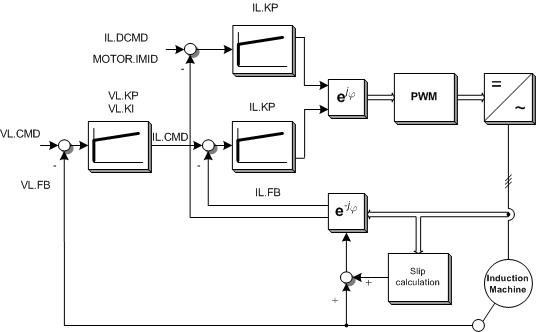Figure 1: Field Oriented Control of an ASM

The velocity command can be obtained from three different sources, depending on DRV.CMDSOURCE

• 0: Service
• 1: Field bus
• 3: Analog

Initial settings

 DRV.OPMODE = 1 Velocity  mode MOTOR.TYPE = 4 V/Hz speed control FB1.SELECT = XX Select according to your feedback DRV.ACC & DRV.DEC Set according to induction machine mechanical dynamics

Parameters

The following table shows which MOTOR parameters should be also configured for driving induction machines under FOC closed-loop operation:

 Keyword Induction Motor Closed Loop Description MOTOR.NAME Yes Set a name for customer motor MOTOR.TYPE Yes Set to motor type. For V/Hz, MOTOR.TYPE = 4 MOTOR.AUTOSET Yes Available for FOC MOTOR.IPEAK Yes Set to motor peak current (A).  150% of continuous current for NEMA motors MOTOR.ICONT Yes Set to motor continuous current (A) MOTOR.INERTIA Yes Set the motor inertia in kg cm2 MOTOR.KT No No necessary for Induction Motor MOTOR.LQLL Yes Set the stator inductance in mH. For further information see below section “calculation of machine parameters”. MOTOR.POLES Yes Set to motor poles MOTOR.VMAX Yes This is the maximum mechanical speed of the motor in RPM MOTOR.R Yes Set the stator winding resistance phase-to-phase in Ohms. For further information see below section “calculation of machine parameters”. MOTOR.VOLTMAX Yes Motor max winding voltage.  Ex: 230 VAC or 460VAC MOTOR.PHASE No No necessary for Induction Motor MOTOR.CTF0 Yes This is used to configure the thermal constant of the motor coil MOTOR.KE No No necessary for Induction Motor MOTOR.IMTR Yes Set the rotor time constant in ms. For further information see below section “calculation of machine parameters”. MOTOR.IMID Yes Set the magnetizing current (id) in A. For further information see below section “calculation of machine parameters”. MOTOR.VOLTRATED No No necessary for FOC MOTOR.VRATED No No necessary for FOC MOTOR.VOLTMIN No No necessary for FOC

Field weakening.

So far, the closed-loop control implemented in AKD is not able to drive the ASM in field weakening area.

Calculation of machine parameters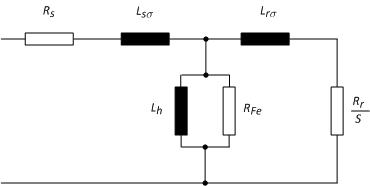Figure 2: Induction motor equivalent circuit

1. Determine current set point id = MOTOR.IMID

The direct-axis (non-torque axis) current Id is set to a constant value as soon as the drive is enabled. MOTOR.IMID is used to set up and view the magnitude of Id set point.

The appropriate value of MOTOR.IMID can be estimated from the motor nameplate information.

A rule of thumb as follows can be used (see S300 manual):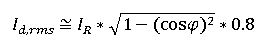1

In which Id is the value for MOTOR.IMID, the preset rotor flux building current. IR and cos(phi)are the phase current and power factor at rated operation, read from the motor data sheet respectively. And 0.8 is an empirical factor. MOTOR.IMID is in Arms.

2. Rotor resistance

The rotor resistance can be calculated based on the electrical motor losses. This means, based on the difference between ideal mechanical power and real mechanical power, the rotor resistance can be calculated as follows: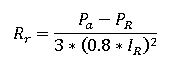2

Where Pa is the ideal mechanical power, N0 is the synchronous speed and TeR is the nominal electromagnetic torque defined as: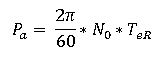3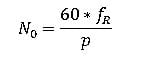4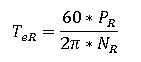5

PR, IR, fR and NR are the nominal values for power, current, frequency and speed, read from motor data sheet, respectively.

3. Rotor inductance

The rotor inductance is defined as LR = Lm + L. Since Lm is bigger than L, we consider that: Lr = Lm. The mutual inductance can be calculated as follows: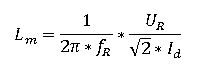6

4. Rotor time constant

Finally, the rotor time constant is calculated as follows: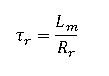7

Vector current limiting

1. Id limits:

• Upper limit: 93.75% fold-back current limit, which is calculated as the smallest of drive current limit, motor current limit, and what fold back algorithm allows.
• Lower limit: -93.75% fold-back current limit.

2. Iq limits:

• Upper: the lower of
• Sqrt(Ifold^2 – Id^2)
• User set Iq positive limit
• Lower: the higher of
• Sqrt(Ifold^2 – Id^2)
• User set Iq negative limit

Commutation angle setting

For induction motor closed loop control the commutation angle is set as shown in the following figure: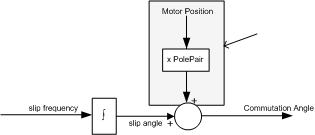Figure 3. Commutation angle setting in AKD for IM

Induction motor close loop control supports using FB1 or FB2 as commutation feedback source.  The shaded calculation, adding motor position feedback to the slip, happens in the FPGA if using FB1 IL.FBSOURCE 0; and in firmware if IL.FBSOURCE is 1

Variables for analysis

Following variables are available for analysis and supervision:

 IL.CMD Currently reference for iq (torque-generation current) IL.FB Currently current for iq, at motor terminals IL.IDCMD Currently reference for id (flux-generation current) VL.CMD Velocity reference VL.FB Shaft position, read from the feedback

Further Information

Such concept is also implemented for our Servostar amplifier. You can find more information in the following links:

Induction Machine - General

Sensorless Control for Induction Machines with S300-S700

Operating Induction Machines S300-S700

Example

Considering the following ASM:

 Nominal voltage = 230/460V Nominal current = 8.75/5.05A 4 poles Power: 2.20Kw Nominal speed = 1395 rpm cos(phi) = 0.82 Nominal frequency = 50Hz

With a resolver as feedback

Then, the missed machine parameters are calculated as follows:

1. MOTOR.IMID:  based on equation 1: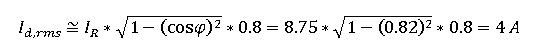1. Rotor resistance.  The nominal electromagnetic torque is calculated based on eq. 5: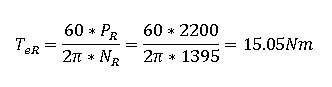Based on eq. 4, we got the synchronous speed as: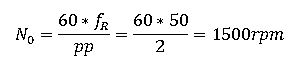The ideal mechanical power from eq. 3 is: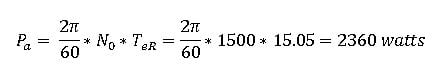From eq. 2, the rotor resistance is then calculated as: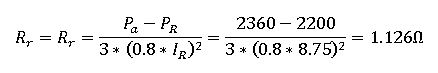1. Mutual inductance. From eq. 6, we got: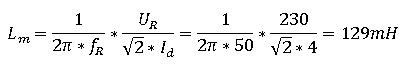1. Rotor time constant. Finally from eq. 7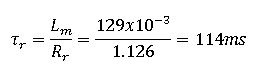We set following parameters in AKD

 Parameter Value Parameter Value DRV.ACC & DRV.DEC 10000rpm/s MOTOR.POLES 4 DRV.OPMODE 1 MOTOR.VMAX 1600 rpm FB1.SELECT 40 MOTOR.R 1.126 ohm MOTOR.NAME NORD-ASM MOTOR.VOLTMAX 240V MOTOR.TYPE 4 MOTOR.IMTR 114 ms MOTOR.IPEAK 13 A MOTOR.IMID 4 A MOTOR.ICONT 8.75 A MOTOR.INERTIA 6.2 kg cm2 MOTOR.LQLL 129 mH

Speed command: 1395 rpm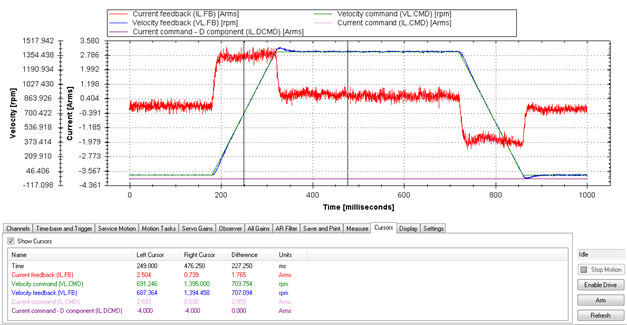AttachmentASM Closed loop FOC AKD - Article.pdf